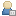Show Posts

This section allows you to view all posts made by this member. Note that you can only see posts made in areas you currently have access to.

Topics - Havard

Pages: 
1
Feedback / Gernot have you seem this...
« on: 2009-Aug-06 »
http://www.shootemupcreator.com/

Just stumbled upon this - no demo or demos to download though....

I'm sure yours is better.2
IDE/Syntax / Folding of Functions in the IDE
« on: 2009-Aug-06 »
Can you or would it be possible to make it so you can fold functions in the IDE - just giving the title of the funciton.  ONce you have a working function it could then be compressed - this makes your code much easier to read.  Have used this before in another basic IDe and it was really helpful as I find following code can be really hard as it grows.

3
2D / Support Fullscreen at lower resolutions or make windows scalable
« on: 2009-Aug-06 »
Title says it all really.  For retroremakes it would be helpful to support fullscreen at 320x200, 320x240 etc

or

Could you make windows scalable so that they can be dragged to any size on the desktop?

4
GLBasic - en / Help for a beginner
« on: 2009-Aug-06 »
Hi

Having frequented retroremakes for a number of years there are a number of familiar names on this site. - so hello old friends!  Having been a Blitz devotee for a number of years, I took up Gernots's offer of an education licence for my school and have been experimenting with GL basic.  I converted one of my games over without too much trouble and now am reviving an old project - Target Renegade Remix - by starting from scratch and rewriting/reusing old code.  I have hit two problems and both of them are quite major and probably linked to my understanding of GL basic.

1. Fullscreen - I can't run at fullscreen or expand teh window to fullscreen at a resolution of 320x200.  Can go fullscreen at 320x240?  This works in blitz.  Any ideas?

2. I am trying to detect simultaneous button presses for cntrl and an arrow key to produce a back kick but I just can't get it to work.  The code is included below - any suggestions, please let me know (I tried a different key instead of cntrl as I wondered if it was a hardware problem with the keyboard but this made no difference).

Code: GLBasic [Select]
// --------------------------------- //
// Project: TR Remix
// Start: Monday, July 27, 2009
// IDE Version: 6.164

SETSCREEN 640,480,TRUE

//player1
LOADANIM "graphics/p1hit.png",102,56,15 //p1 hit to floor
LOADANIM "graphics/p1flyingkick.png",103,40,46 //flying kick
LOADANIM "graphics/p1floorpunch.png",105,28,30 //p1 floor punch
LOADANIM "graphics/p1knee.png",106,32,42 //p1 knee
LOADANIM "graphics/p1bk.png",107,40,45 //p1 back kick

//Set up variables
GLOBAL p1x=100,p1y=60,p1f=0,p1dir=1,p1state=0
GLOBAL scroll=0,lives=3

//main loop
WHILE KEY(57)=0

//draw background
DRAWSPRITE 0,n,0
DRAWSPRITE 1,0,141
FOR n=1 TO lives;       DRAWSPRITE 99,n*20,170;         NEXT
//PRINT "P1",0, 150
//a\$=Str(p1score): ss=LEN(a\$): a\$="":FOR n=0 TO 5-ss: a\$=a\$+"0":NEXT: a\$=a\$+Str(p1score)
//jf_text (fntzx16, 25, 150, a\$)
//jf_text (fntzx16, 205, 150, "P1")

keypress()
drawsprites()
SHOWSCREEN

WEND

FUNCTION keypress:

right1=0; left1=0; up1=0; down1=0;

IF KEY(205) THEN right1=1
IF KEY(203) THEN left1=1
IF KEY(200) THEN up1=1
IF KEY(208) THEN down1=1

//code to make player release punch key
IF KEY(29) THEN b1=1
IF KEY(29)=0 THEN b1=0
IF b1=1 AND bd=0 THEN punch1=1
bd=b1

PRINT "punch="+punch1,100,150
PRINT "right="+right1,100,160
PRINT "left="+left1,100,170
PRINT "up="+up1,100,180
PRINT "p1state="+p1state,100,190
PRINT "p1dir="+p1dir, 20,150
PRINT "bd="+bd, 20,160
PRINT "b1="+b1, 20,170

IF p1state<>0 THEN GOTO jump2

//check for back kick
IF punch1 AND right1 //AND p1dir=-1
p1state=2
p1f=0
delay=0
ENDIF

IF punch1 AND left1 //AND p1dir=1
p1state=2
p1f=0
ENDIF

//check for just a punch
IF punch1=1 AND p1state=0
p1state=1
p1f=0
ENDIF

IF right1=1
p1x=p1x+1
p1dir=1
p1f=p1f+.3
ENDIF

IF left1=1
p1x=p1x-1
p1dir=-1
p1f=p1f-.3
ENDIF

IF up1=1
p1y=p1y-1
p1f=p1f+(p1dir*.3)
ENDIF

IF down1=1
p1y=p1y+1
p1f=p1f+(p1dir*.3)
ENDIF

IF p1f>11 THEN p1f=0
IF p1f<0 THEN p1f=10
//limits fo x AND y movement FOR player
IF p1x<8 THEN p1x=8
IF p1x>300 THEN p1x=300
IF p1y>92 THEN p1y=92
IF p1y<42 THEN p1y=42

//scroll screen if all baddies beaten in section
IF p1x>200 AND scroll=1
p1x=200
n=n-1
ENDIF

jump2:

ENDFUNCTION

// 0rder sprites from top to bottoom
FUNCTION drawsprites:
FOR n=42 TO 92
IF p1state<>0 THEN GOTO JUMP1

IF p1dir=1 AND p1y=n
DRAWSPRITE 101,p1x,p1y+35
DRAWANIM 100,p1f,p1x,p1y
ENDIF

IF p1dir=-1 AND p1y=n
DRAWSPRITE 101,p1x+6,p1y+35
DRAWANIM 100,p1f+12,p1x,p1y
ENDIF

JUMP1:
//punch
IF p1state=1 AND p1dir=1 AND p1y=n
DRAWSPRITE 101,p1x,p1y+35
DRAWANIM 104,p1f,p1x-6,p1y-3
p1f=p1f+.1

IF p1f>3
p1state=0
p1f=0
punch1=0
ENDIF

ENDIF

IF p1state=1 AND p1dir=-1 AND p1y=n
DRAWSPRITE 101,p1x+6,p1y+35
DRAWANIM 104,p1f+7,p1x-4,p1y-3
p1f=p1f+.1

IF p1f>3
p1state=0
p1f=0
punch1=0
ENDIF
ENDIF

//back kick
IF p1state=2 AND p1dir=1 AND p1y=n
DRAWSPRITE 101,p1x,p1y+35
DRAWANIM 107,p1f+2,p1x-12,p1y-3
p1f=p1f+.1; IF p1f>1 THEN p1f=1
INC delay, 1

IF p1f=1 AND delay=50
p1state=0
p1f=0
punch1=0
delay=0
ENDIF

ENDIF

IF p1state=2 AND p1dir=-1 AND p1y=n
DRAWSPRITE 101,p1x+4,p1y+35
DRAWANIM 107,p1f,p1x+6,p1y-3
p1f=p1f+.1; IF p1f>2 THEN p1f=2

IF p1f=2 AND delay=50
p1state=0
p1f=0
punch1=0
delay=0
ENDIF

ENDIF
NEXT

ENDFUNCTION

If you want to check the old blitz version then click below: